# Polynomial regression with known variance of classical error

3Polynomial regression with known variance of classical error

In Chapter 1, it was mentioned that the binary observation model (1.2), (1.4), and (1.5) is widespread in radio-epidemiology. This binary model is the so-called generalized linear model (GLM). This means that the conditional distribution of the response y given the true value of regressor ξ is expressed through a linear function in ξ, with some unknown parameters. The linear function defines the odds function (1.4). For more information on the generalized linear models, see Chapter 4.

The model (1.2), (3.1), and (1.5) serves for modeling the thyroid cancer incidence as a result of exposure by radioactive iodine: ξi is a radiation dose received by a subject i from a cohort during a fixed observation period. Positive radiation risk parameters β0, β1, and β2 are to be estimated. The presence of a negative term member in the quadratic odds function (3.1) describes the effect of burning out cancer cells at high exposure doses, which may even lead to some reduction of disease incidence.

The binary regression model (1.2) and (3.1) is no longer the GLM model, because now the conditional distribution of yi given ξi is expressed through the quadratic (3.1), but not through a linear function in ξi.

To get a feeling for the effect of polynomial influence of regressor on response, consider the polynomial regression model with classical measurement error. It is described by the two equations:

Here, k ≥ 1 is a given degree of the polynomial (at k = 1 we get the linear model from Chapter 2), ξi is a latent variable, xi is an observed surrogate data, yi is an observed response, εi and δi are observation errors. The regression parameter

has to be estimated.

Introduce the vector function

Now, equality (3.2) can be rewritten in the compact form

We consider the structural model in which the ξi are random variables. Make the following assumptions about the observation model.

(ii)The errors εi are identically distributed with distribution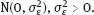(iii)The errors δi are identically distributed with distribution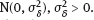(iv)Random variables ξi are identically distributed with distribution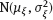,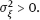The model (3.2) and (3.3), with assumptions (i)–(iv), is called normal structural polynomial model with classical measurement errors. Such models can be used, e.g., in econometrics (Carroll et al., 2006).

Section 2.3 states that at k = 1 without additional assumptions about the parameters, this model (in this case being linear) is not identifiable. From here, it follows as well that at k ≥ 2, the normal polynomial model is not identifiable, because in the proof of Theorem 2.10 for the polynomial model, an additional condition for the rest of the true parameters can be imposed:

In the absence of further restrictions, the normal polynomial model is determined by a vector parameter θ and the corresponding parameter set Θ:

Let it be assumed additionally that k ≥ 2 and condition (3.7) is violated, i.e., the true regression function

If we allow the degeneracy (3.7), then we need some restriction of the parameter set (3.8) to get the identifiability of the model. In this section, it is required that the measurement error variance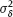is known.

Rarely, the ratio of error variances

is assumed known. In Shklyar (2008), consistent estimators of model parameters are constructed for this case.

Note that for k ≥ 2, the ML method in the normal model with assumptions (i)–(v) is not feasible (see discussion in Section 1.4.2). In particular, even under known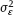(thenis known as well as a result of (v)) the joint pdf of the observed variables y and x is given by the integral (1.54), with the polynomial regression function f(ξ) = f(ξ, β) as defined in (3.9). The integral is not calculated analytically, which complicates the usage of the ML method and makes it problematic to study properties of the estimator.

## 3.1The adjusted least squares estimator

### 3.1.1The formula for the estimator

The corrected score method was described in Section 1.4.4. Apply it to the model (3.6) and (3.3). The regression function is

Within the class of polynomials in ξ, the solutions are unique:

where the vector function t(x) and matrix-valued function H(x) satisfy the deconvolution equations

Hereafter, all the equalities for conditional expectations hold almost surely (a.s.). For the jth component of the function t(x), it holds that

For an entry Hij(x) of the matrix H(x), we have

Below we show that deconvolution equation (3.16) has a unique polynomial solution tj(x), and then

A solution to equation (3.16) is given by the Hermite polynomial Hj(x) closely related to normal distribution. The polynomial can be specified by an explicit formula through higher derivatives:

In particular,

Applying the formula for j = 2 and j = 3, we get

The recurrence relation (3.21) allows us to compute consequently the next Hermite polynomials; all of them have unit leading coefficient. Let

Then for all n, m ≥ 0, the equality holds:

Here, δnm is the Kronecker symbol:

The next Hermite polynomial property is due to Stulajter (1978). We give a simple proof.

Proof. We use induction. Denote

and (3.26) holds true. For n = 1, we have, see (3.20):

and (3.26) is fulfilled as well.

(b)Derive a recurrence relation for expressions (3.27). If n ≥ 1, we have in view of the fact that μ + γ ∼ N(μ,1):

Using equation (3.30) and identity (3.21), with j = n + 1, further we get

(c)Assume that (3.26) holds true, for all nk, where k ≥ 1 is fixed. Then from equation (3.33), we will have

Thus, we obtain (3.26), with n = k + 1.

According to the method of mathematical induction, (3.26) has been proved for all n ≥ 0, with μR.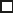Corollary 3.2. In case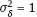, equality (3.16) is valid, with tj(x) = Hj(x).

Proof. By equality (3.26), it follows (now, both ξ and γ are independent and γ ∼N (0, 1)) that:

which proves the desired statement.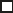which proves the statement of the lemma.As we can see, the function (3.36) is the only solution to the deconvolution equation (3.16) in the class of polynomials in ξ. Now, construct the estimating function (1.126) by means of the ALS method:

The ALS estimator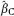is found from the equation

(v)Random variables ξi are identically distributed, with E(ξ1)2k < ∞; moreover, the distribution of ξ1 is not concentrated at k or even fewer points.

The latter requirement about the distribution means the following: for each set {a1, …, ak} ⊂ R,

Proof. From (3.15), using the SLLN, we obtain (here x =d x1):

The limit matrix is the Gram matrix for random variables 1, ξ ,…, ξk in the space L2(, P) of random variables on having finite second moment. In this space, an inner product is

This Gram matrix is nonsingular if, and only if, the random variables 1, ξ ,…, ξk are linearly independent in L2(, P). Prove that the latter holds.

Suppose that for some real numbers a0, …, ak, we have

Then, ξ coincides almost surely with some root of the polynomial p (z) = a0 + a1z + … akzk. If not all coefficients of the polynomial are zeros, then the polynomial has no more than k real roots, and therefore, ξ almost surely belongs to the set of roots. Thus, we got a contradiction to condition (v) about the distribution of ξ.So a0 = a1 = ··· = ak = 0 proving the linear independence of 1, ξ ,…, ξk.

Thus, eventually the matrix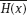is nonsingular.

Lemma 3.4 shows that under conditions (iii) and (v), the ALS estimator is eventually given by formula (3.40). In particular, in the case (iv), ξ has a continuous distribution and then condition (v) holds true.### 3.1.2Consistency of the estimator

The ALS estimator remains strongly consistent without the assumption on normality of errors εi and regressors ξi. Introduce a weaker assumption.

(vi)The errors εi are centered and identically distributed.

Proof. Use equality (3.40) that holds eventually.

By the SLLN and the first equality in (3.15), we have

The condition (v) holds. Thus, according to (3.45), the matrix EρρT is nonsingular. In equation (3.40), let us tend n to infinity:

The proof is accomplished.### 3.1.3Conditional expectation and conditional variance of response

To ensure asymptotic normality of the estimator, we need the existence of the second moment of errors εi. Assume the following.

(vii)The errors εi are centered with variance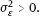We use relations (1.86) and (1.97). Denote

May 26, 2018 | Posted by in NUCLEAR MEDICINE | Comments Off on Polynomial regression with known variance of classical error
Premium Wordpress Themes by UFO Themes